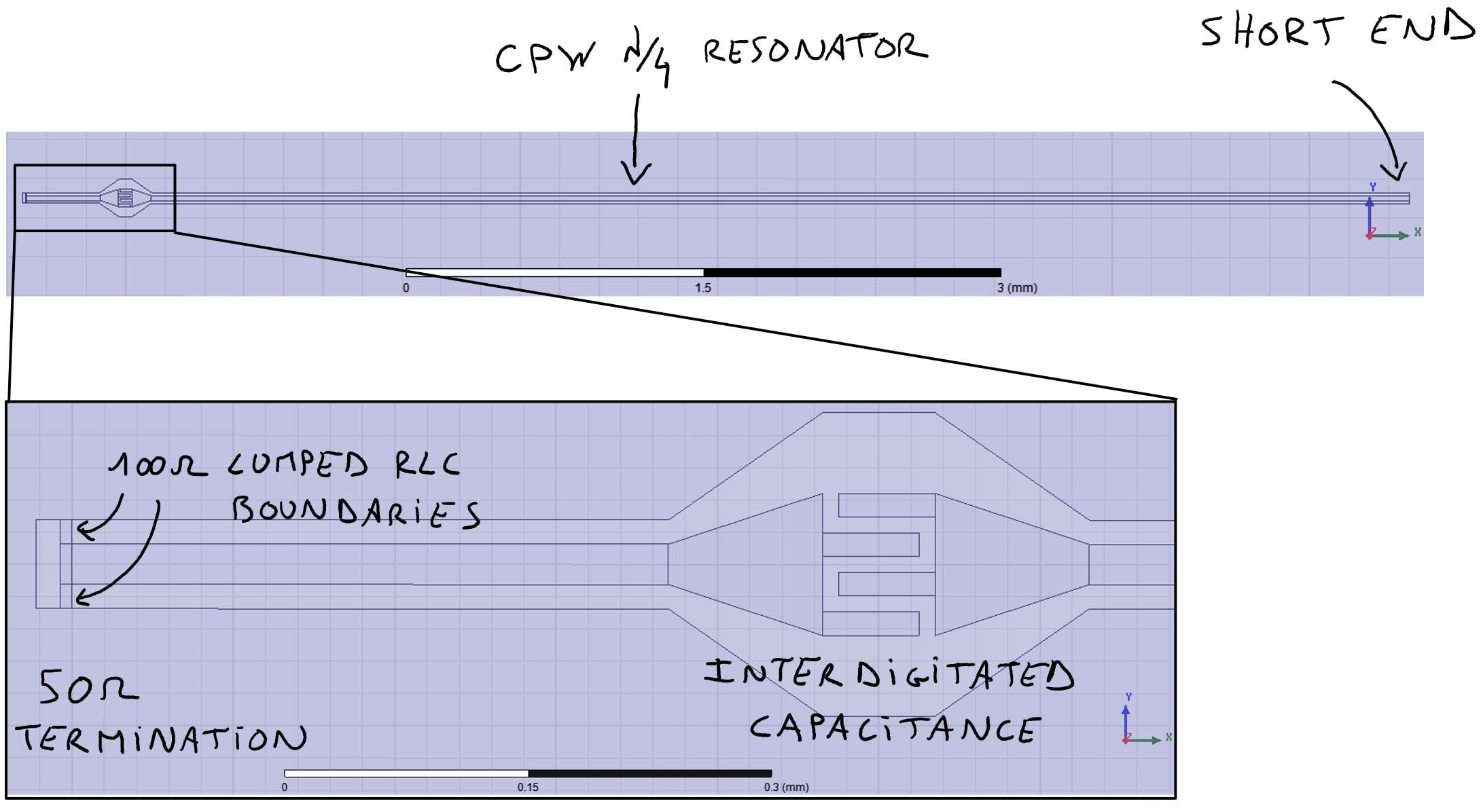# Inconsistent loss calculations with HFSS field calculator

Hi everyone,

I hope you're doing well even if you are in self isolation.

I observe some inconsistencies when using the HFSS field calculator to calculate losses in a circuit which I need to understand.

Let me reduce my problem to a very simple case : an eigenmode simulation of a quarter-wavelength CPW resonator. The resonator is shorted at one end and capacitively coupled to a 50ohm termination at the other end. See below.The eigenmode solver finds a resonant mode at frequency f=8.531 GHz and quality factor Q=10,727.7. So far so good.

In this simulation, the substrate (silicon) is lossless and the conductors are sheets with PerfectE boundaries. So the only lossy element is the 50ohm termination. It is made with 2 parallel 100 ohm sheets with Lumped RLC boundaries (R=100ohm, L=0, C=0), see above picture.

My problem happens when I try to calculate the quality factor using the HFSS field calculator (it is useless in this simplified case, but not in my actual simulations) : 2 methods that should give the exact same results give different results.

Method 1
First I calculate the total dissipated power by integrating the SurfaceLossDensity on each 100 ohms resistors (named R_up and R_dn). The formula is :
P1 = Integrate(Surface(R_up), SurfaceLossDensity) + Integrate(Surface(R_dn), SurfaceLossDensity),
from which I deduce the quality factor :
Q1 = 2 * pi * f * E / P1,
where E = 1 Joule is the mode energy.

Method 2
I integrate directly the real part of the Poynting vector normal to each 100 ohms resistors. The formula is :
P2 = Integrate(Surface(R_up), Dot(Real(Poynting), SurfaceNormal)) + Integrate(Surface(R_dn), Dot(Real(Poynting), SurfaceNormal)),
from which I deduce the quality factor :
Q2 = 2 * pi * f * E / P2

The 2 methods should be exactly identical, but I get :
Q1 = 10,727.7
Q2 = 23,095.8
[Reminder : Q = 10,727.7]

Then I wondered if some factor 2 was missing in Method 2 because the sheets have 2 faces, however :
Q2 / 2 = 11,547.9

Why method 2 is giving such a different result ? How can I fix it ? It is very important to me because in some cases my Lumped RLC is an inductor and I need to calculate the magnetic energy stored in it. Then I integrate the imaginary part of the Poynting vector. But since method 2 fails for the real part of the Poynting vector, I'm worried I get wrong results.

Sébastien

• Hello, while I am not able to solve your problem, I can add that I also see a difference in the calculation. I only need to calculate the surface power lost (Watts), so I calculate what you call P1 and P2. We both have the same case where P1 is kinda of close to half of what P2 is. My P1 is be 73.698 and my P2 is 45.356. I am not sure where the difference comes from.

• Hello,

We have reached out internally to the right expert and we should be able to respond to this soon.

Thank you for your patience with this.

Best,

Karthik